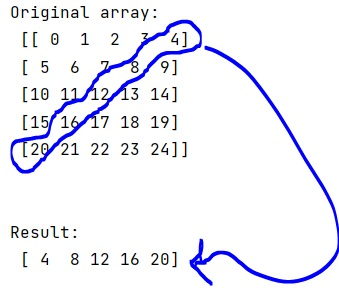# How to get the opposite diagonal of a NumPy array?

Given a NumPy array, we have to get the opposite diagonal from it.
Submitted by Pranit Sharma, on March 12, 2023

## Getting the opposite diagonal

Diagonals of an array are defined as a set of those points where the row and the column coordinates are the same.

Suppose that we are given a multi-dimensional numpy array and we need to get the opposite diagonals of this array. By this, we mean that we need to get the diagonal starting from the top right rather than the top left. Numpy has a built-in function for the diagonal indices but there is no direct option to get the opposite diagonals.

To get the opposite diagonal, we can simply use the numpy.rot90() function to rotate the numpy array with 90 degrees and then we can apply the numpy.diag() function on the rotated array.

Let us understand with the help of an example,

## Python code to get the opposite diagonal of a NumPy array

```# Import numpy
import numpy as np

# Creating a numpy array
arr = np.arange(25).reshape(5,5)

# Display Original array
print("Original array:\n",arr,"\n\n")

# Rotating the array
rot = np.rot90(arr)

# Getting opposite diagonals
res = np.diag(rot)

# Display result
print("Result:\n",res)
```

### OutputLanguages: » C » C++ » C++ STL » Java » Data Structure » C#.Net » Android » Kotlin » SQL
Web Technologies: » PHP » Python » JavaScript » CSS » Ajax » Node.js » Web programming/HTML
Solved programs: » C » C++ » DS » Java » C#
Aptitude que. & ans.: » C » C++ » Java » DBMS
Interview que. & ans.: » C » Embedded C » Java » SEO » HR
CS Subjects: » CS Basics » O.S. » Networks » DBMS » Embedded Systems » Cloud Computing
» Machine learning » CS Organizations » Linux » DOS
More: » Articles » Puzzles » News/Updates